#### 期刊菜单

AZ31镁合金板材压弯–轧平复合形变组织性能研究
Study of Composite Deformation Properties of AZ31 Magnesium Alloy Sheet Bending-Rolling Flat
DOI: 10.12677/MEng.2022.94034, PDF , HTML, XML, 下载: 72  浏览: 119  科研立项经费支持

Abstract: In order to investigate the effect of bending-rolling composite deformation of magnesium alloy on its organization, by constructing macroscopic finite element model and microscopic dynamic recrystallization model, the multi-directional bending-rolling composite deformation of AZ31 magnesium alloy with different thicknesses was simulated at 400˚C, and examined the change of its weave. The results show that: the equivalent stress increases with the number of passes and decreases with the thickness of the plate, and the peak stress value is larger than the trough; the recrystallization phenomenon is more obvious with the increase of deformation passes, and twinning occurs during the deformation process, and the proportion of twinning increases in two passes. The microstructure changes in the composite deformation of AZ31 magnesium alloy were simulated by the cellular automata method (CA), and the microscopic simulation results were consistent with the experiments. The composite deformation can effectively weaken the basal weave of the plate as shown by EBSD inspection. By establishing the microscopic simulation model of composite deformation of AZ31 magnesium alloy, it is able to simulate the process of composite deformation of press bending and rolling flat, which is a certain guidance for composite deformation of magnesium alloy.

1. 引言

2. 实验

2.1. 实验方法Table 1. Chemical composition of AZ31 magnesium alloy (mass fraction/%)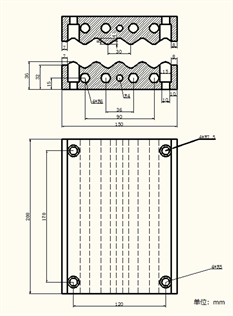(1) 模具二维图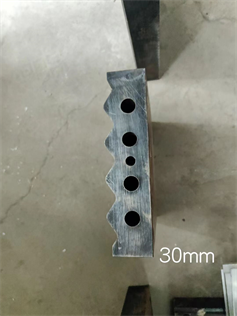(2) 模具实物图

Figure 1. Press-bending tooling diagramTable 2. Basic performance parameters of four column hydraulic press

2.2. 有限元模型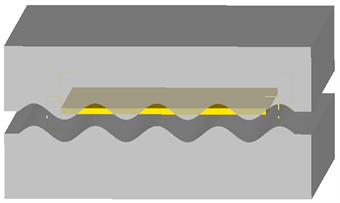(a) 压弯变形三维模型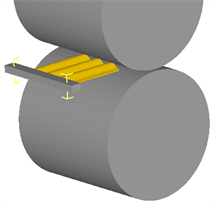(b) 轧制变形三维模型

Figure 2. Mold and plate 3D model

$\stackrel{˙}{\epsilon }=A{\left[\mathrm{sin}\left(\alpha \sigma \right)\right]}^{n}\mathrm{exp}\left[-Q/\left(RT\right)\right]$ (1)

2.3. 微观组织模型

AZ31镁合金材料微观组织模拟需要的相关参数见表4Table 4. AZ31 magnesium alloy material parameters

2.3.1. 元胞自动机

2.3.2. 位错密度模型

$\text{d}{\rho }_{i}=\left(h-r{\rho }_{i}\right)\text{d}\epsilon$ (2)

$r={r}_{0}{\left(\frac{\stackrel{˙}{\epsilon }}{{\stackrel{˙}{\epsilon }}_{0}}\right)}^{-m}\cdot \mathrm{exp}\left(\frac{-mQ}{RT}\right)$ (3)

$h={h}_{0}{\left(\frac{\stackrel{˙}{\epsilon }}{{\stackrel{˙}{\epsilon }}_{0}}\right)}^{-m}\cdot \mathrm{exp}\left(\frac{mQ}{RT}\right)$ (4)

$r=17.7{\stackrel{˙}{\epsilon }}^{-0.2}\cdot \mathrm{exp}\left(\frac{-0.2Q}{8.314T}\right)$ (5)

$h={10}^{13}{\stackrel{˙}{\epsilon }}^{-0.2}\cdot \mathrm{exp}\left(\frac{0.2Q}{8.314T}\right)$ (6)

2.3.3. 再结晶形核模型

$\stackrel{˙}{n}=C{\stackrel{˙}{\epsilon }}^{m}\mathrm{exp}\left(\frac{-{Q}_{D}}{RT}\right)$ (7)

2.3.4. 晶粒长大模型

${v}_{i}=\frac{b}{KT}D\mathrm{exp}\left(\frac{-Q}{RT}\right){F}_{i}/\left(4\pi {r}_{i}^{2}\right)$ (8)

${F}_{i}=4\pi {r}_{i}^{2}\tau \left({\rho }_{m}-{\rho }_{i}\right)-8\pi {r}_{i}{\gamma }_{i}$ (9)

3. 结果分析

3.1. 应力分析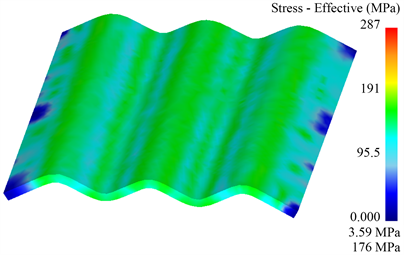(a) 压弯过程中应力场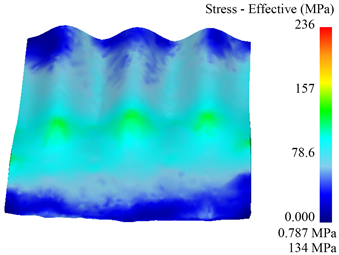(b) 轧平过程中应力场

Figure 3. Stress field distribution during deformation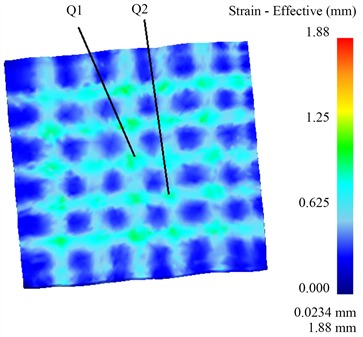Figure 4. Feature point selection location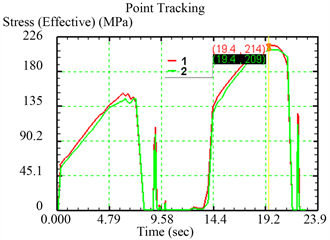(a) 轧制垂直与压弯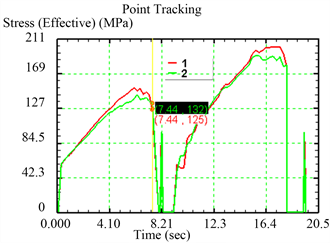(b) 轧制平行于压弯

Figure 5. Thickness of 3 mm plate two times the composite deformation equivalent force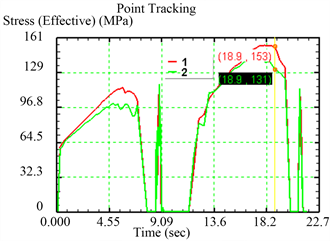(a) 轧制垂直与压弯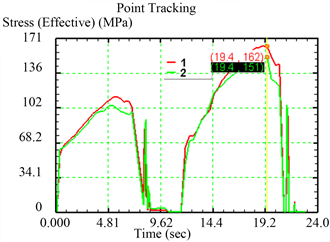(b) 轧制平行于压弯

Figure 6. Thickness of 5 mm plate two times the composite deformation equivalent forceFigure 7. TC rimping contact diagramTable 5. Maximum equivalent force values for different thicknesses of plates, rolling directions, and passes (unit: MPa)

3.2. 微观结果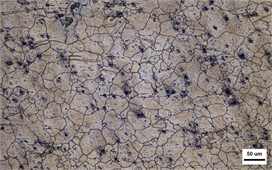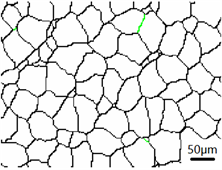(a) 原始组织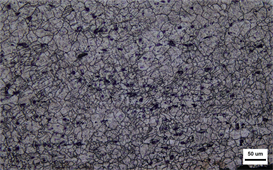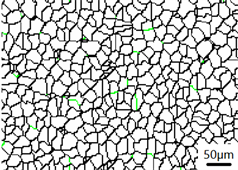(b) 轧制垂直于压弯单道次变形组织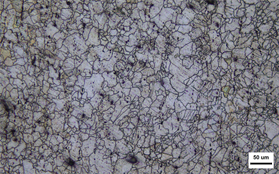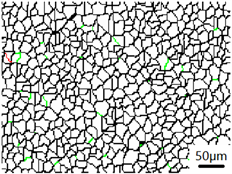(c) 轧制平行于压弯单道次变形组织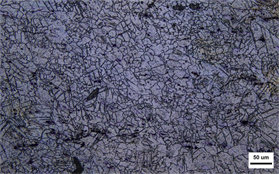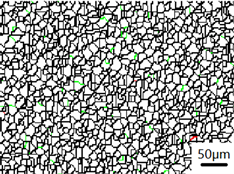(d) 轧制垂直与压弯两道次复合形变组织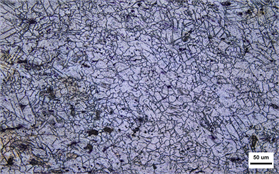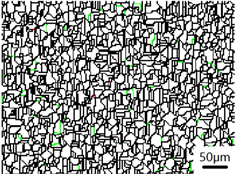(e) 轧制平行于压弯两道次复合形变组织

Figure 8. Comparison of simulated and actual organizationTable 6. Average grain size of simulated and actual tissues under different processes

3.3. 织构分析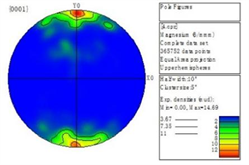(a) 原始板材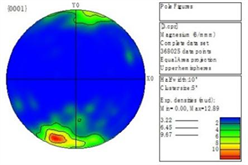(b) 单道次轧平垂直与压弯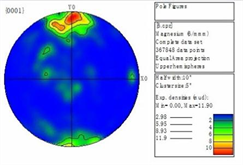(c) 单道次轧制平行于压弯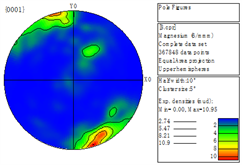(d) 双道次轧制垂直于压弯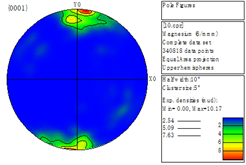(e) 双道次轧制平行于压弯

Figure 9. Pole diagram under different processes

4. 结论

1) 所选特征点第二道次的应力值大于第一道次，波峰处应力值略小于波谷处，5 mm板材平均应力小于3 mm板材，轧制方向对应力影响不大。

2) 单道次复合形变再结晶形核区域在原始晶粒附近，且存在被拉长的晶粒。单道次复合形变轧制垂直于压弯出现少量孪晶，两道次复合形变均出现孪晶现象，再结晶程度更大，两道次变形平均晶粒尺寸较单道次晶粒尺寸小。

3) 采用元胞自动机法对镁合金板材的微观组织演变进行了数值模拟。模拟结果与实验结果基本相吻合，平均晶粒尺寸的最大相对误差为6.34%。

4) 压弯–轧平复合形变能有效地弱化板材织构，轧平反方向与压弯平行时弱化较明显，两道次弱化程度较大。

  王忠堂, 刘永哲, 梁海成, 等. AZ31镁合金动态再结晶临界应变和稳态应变[J]. 沈阳理工大学学报, 2021, 40(2): 39-43.  Catorceno, L.L.C., De Abreu, H.F.G. and Padilha, A.F. (2018) Effects of Cold and Warm Cross-Rolling on Microstructure and Texture Evolution of AZ31B Magnesium Alloy Sheet. Journal of Magnesium and Alloys, 6, 121-133. https://doi.org/10.1016/j.jma.2018.04.004  于仁杰, 梁海成, 张玉鹏, 等. 挤压态ZK60镁合金棒材的热变形行为[J]. 沈阳理工大学学报, 2021, 40(4): 53-59.  Yu, H.L., Lu, C., Tieu, A.K., Li, H.J., Godbole, A. and Zhang, S.H. (2016) Special Rolling Techniques for Improvement of Mechanical Properties of Ultrafine-Grained Metal Sheets: A Review. Advanced Engineering Materials, 18, 754-769. https://doi.org/10.1002/adem.201500369  金旭. 剧烈塑性变形镁合金微观组织及变形性能研究[D]: [硕士学位论文]. 南京: 南京理工大学, 2020.  刘筱, 朱必武, 李落星, 等. 挤压态AZ31镁合金热变形过程中的孪生和织构演变[J]. 中国有色金属学报, 2016, 26(2): 288-295.  Luo, D., Wang, H.Y., Zhao, L.G., Wang, C., Liu, G.J., Liu, Y. and Jiang, Q.C. (2017) Effect of Differential Speed Rolling on the Room and Elevated Temperature Tensile Properties of Rolled AZ31 Mg Alloy Sheets. Materials Characterization, 124, 223-228. https://doi.org/10.1016/j.matchar.2016.12.007  王忠堂, 杨念, 陈佳鑫, 等. 镁合金筋条式壁板压弯成形研究[J]. 沈阳理工大学学报, 2020, 39(1): 1-5+14.  任国成. AZ31镁合金ECAP挤压过程塑性变形与组织演变规律的研究[D]: [博士学位论文]. 济南: 山东大学, 2013.  支晨琛, 马立峰, 黄庆学, 等. 交叉轧制对AZ31B镁合金边部组织及成形性的影响[J]. 稀有金属材料与工程, 2018, 47(5): 1555-1561.  田静, 邓嘉飞, 常原颖, 梁伟. AZ31镁合金板弯曲矫直过程中的应力分析及组织演变[J]. 热加工工艺, 2023(7): 85-88.  王忠堂, 张士宏, 齐广霞, 等. AZ31镁合金热变形本构方程[J]. 中国有色金属学报, 2008, 18(11): 1977-1982.  Lee, J.H., Lee, J.U., Kim, S.H., Song, S.W., Lee, C.S. and Park, S.H. (2018) Dynamic Recrystal-lization Behavior and Microstructural Evolution of Mg Alloy AZ31 through High-Speed Rolling. Journal of Mate-rials Science & Technology, 34, 1747-1755. https://doi.org/10.1016/j.jmst.2018.03.002  刘筱, 王洋洋, 叶俊宏, 等. AZ31镁合金高应变速率轧制宏微观仿真[J]. 材料导报, 2021, 35(14): 14101-14106+14114.  Mecking, H. and Kocks, U.F. (1981) Kinetics of Flow and Strain-Hardening. Acta Metallurgica, 29, 1865-1875. https://doi.org/10.1016/0001-6160(81)90112-7  Laasraoui, A. and Jonas, J.J. (1991) Prediction of Steel Flow Stresses at High Temperatures and Strain Rates. Metallurgical Transactions A, 22, 1545-1558. https://doi.org/10.1007/BF02667368  Ding, R. and Guo, Z.X. (2001) Coupled Quantitative Simulation of Microstructural Evolution and Plastic Flow during Dynamic Recrystallization. Acta Materialia, 49, 3163-3175. https://doi.org/10.1016/S1359-6454(01)00233-6  王超渊, 东赟鹏, 宋晓俊, 等. 变形温度及变形量对挤压态FGH96合金晶粒异常长大的影响[J]. 航空材料学报, 2016, 36(5): 14-20.